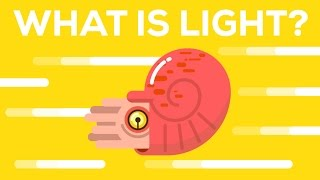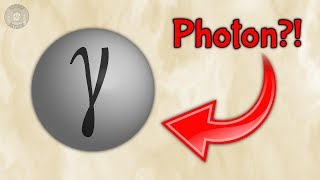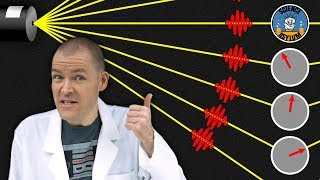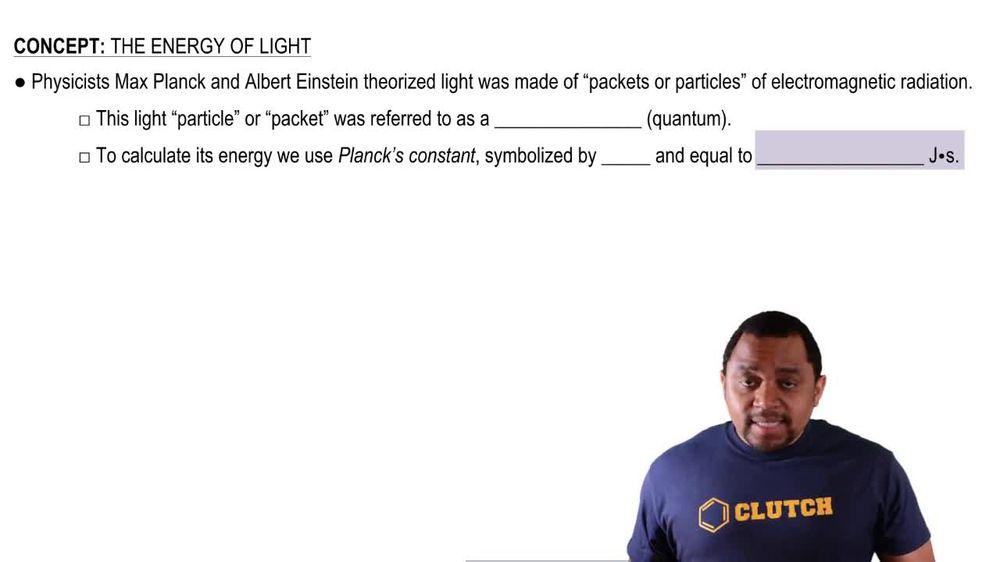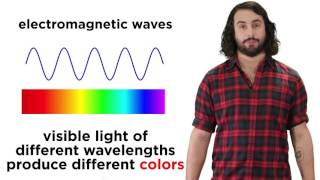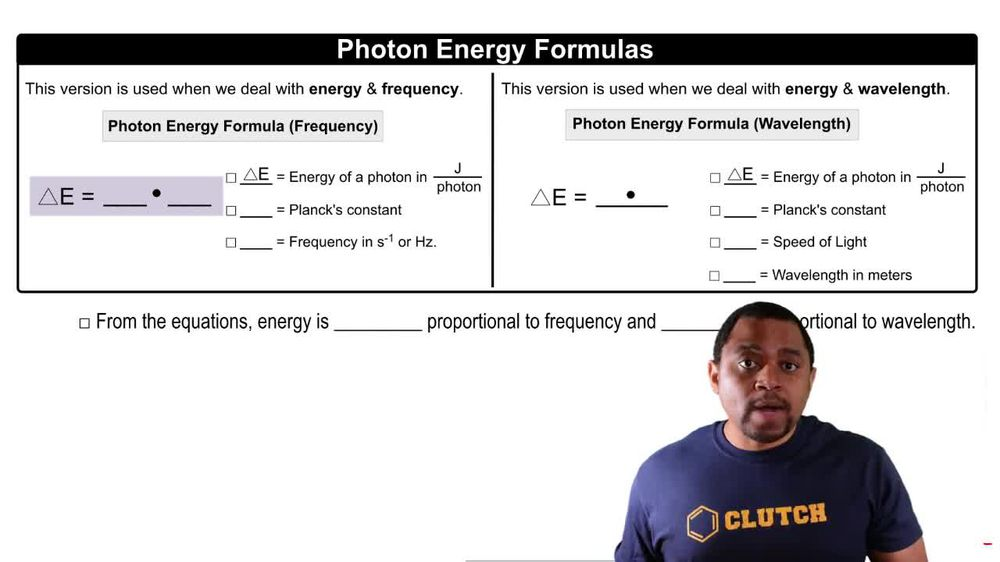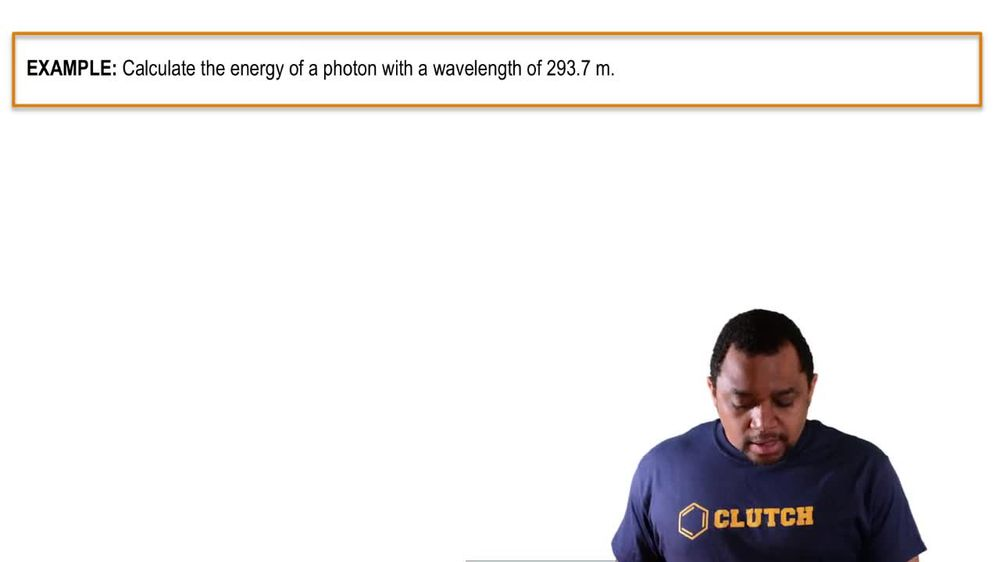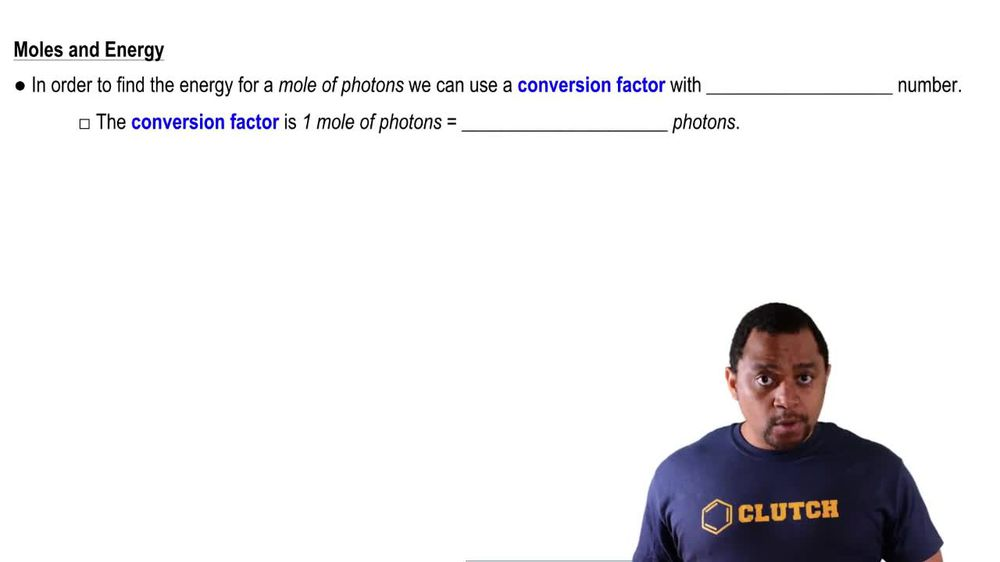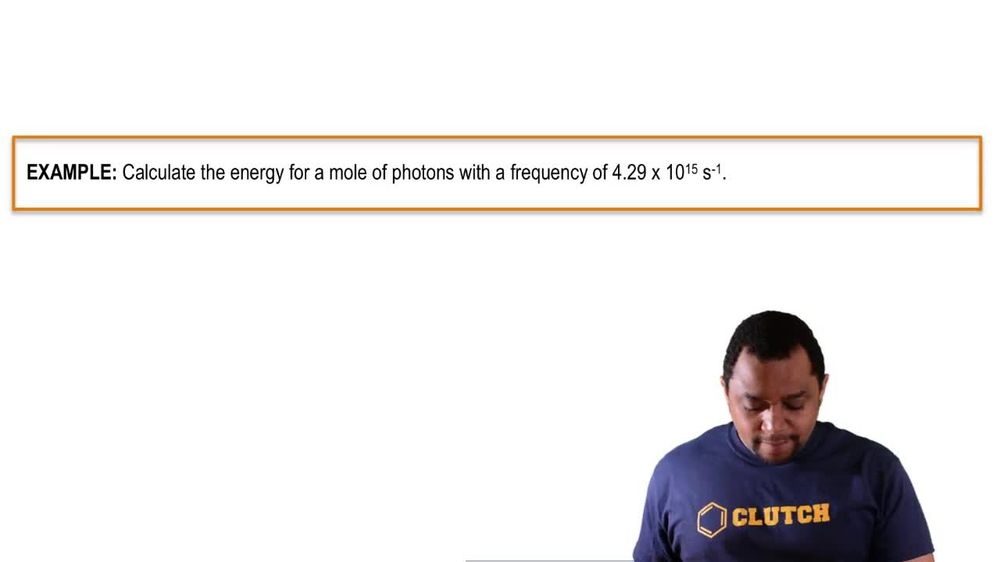Start typing, then use the up and down arrows to select an option from the list.
1. 9. Quantum Mechanics2. The Energy of Light# The Energy of Light Example 2

by Jules Bruno
147 views
3
0
here. It says to calculate the energy for mobile photons with the frequency off 4.29 times 10 to the 15 seconds inverse. All right, so what we're gonna have here is we have energy and they're giving us frequency. So we're gonna say here energy of a photon equals plank's constant times frequency. So that's gonna be 6.626 times 10 to the negative. 34 jewels. Time seconds. That's plank's constant times are frequency in seconds in verse. Okay, so here's seconds we cancel out with inverse seconds when we get initially is 2.84 to 554 times 10 to the negative. 18 jewels per photo. Remember, we don't want to round until the very end. So now that we have jewels per photon realized, we need to find it in terms of moles of photons. So we're gonna use our conversion factor. So we're going to say here that we have, for every one mole of photons, we have 6.22 times 10 to the 23 photons. So here photons cancel out, and I'll have my answer in jewels per mole of photons. So this comes out to 1.7 one times 10 to the six jewels per mole of photons. Okay, so now our answer here has 366 Because our initial value also had 366